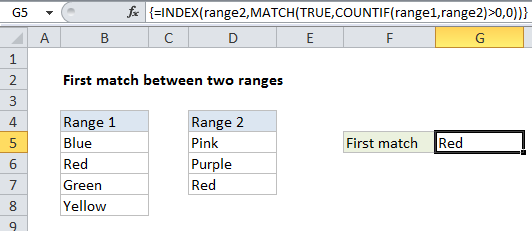## Excel Office

Excel How Tos, Tutorials, Tips & Tricks, Shortcuts

# How to retrieve first match between two ranges in Excel

To retrieve the first match in two ranges of values, you can use a formula based on the INDEX, MATCH, and COUNTIF functions.

## Formula

`=INDEX(range2,MATCH(TRUE,COUNTIF(range1,range2)>0,0))`## Explanation

In the example shown, the formula in G5 is:

`=INDEX(range2,MATCH(TRUE,COUNTIF(range1,range2)>0,0))`

where “range1” is the named range B5:B8, “range2” is the named range D5:D7.

### How this formula works

In this example the named range “range1” refers to cells B5:B8, and the named range “range2” refers to D5:D7. We are using named ranges for convenience and readability only; the formula works fine with regular cell references as well.

Also See:   Find Closest Match in Excel Using INDEX, MATCH, ABS and MIN functions

The core of this formula is INDEX and MATCH. The INDEX function retrieves a value from range2 that represents the first value in range2 that is found in range1. The INDEX function requires an index (row number) and we generate this value using the MATCH function, which is set to match the value TRUE in this portion of the formula:

`MATCH(TRUE,COUNTIF(range1,range2)>0,0)`

Here, the match value is TRUE, and the lookup array is created with COUNTIF here:

`COUNTIF(range1,range2)>0`

COUNTIF returns a count of the range2 values that appear in range1. Because range2 contains multiple values, COUNTIF will return multiple results that look like this:

`{0;0;1}`

We use “>0” to force all results to either TRUE or FALSE:

`{FALSE;FALSE;TRUE}`

Then MATCH does its thing and returns the position of the first TRUE (if any) that appears, in this case, the number 3.

Also See:   Extract multiple matches into separate rows in Excel

Finally, INDEX returns the value at that position, “Red”.